# Colleges with the highest SAT scores in Nebraska

Top 7 colleges in Nebraska with the highest SAT scores
Looking for the colleges with the highest SAT scores in Nebraska? Well you're in luck! We've compiled a national college database and have created a list of the top 7 universities with the highest SAT scores in Nebraska. These are the schools whose applicants had the highest average SAT scores in Nebraska. And, since these tests are meant to determine academic prowess, they are arguably the schools with the most academically proficient students. You could even say these are the best colleges in Nebraska. We also include each college's ACT scores and acceptance rate so that you can see where you would have the easiest or hardest time getting in. Read on to find out more.

## Creighton University SAT scores

The average SAT score for Creighton University is 1254.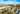The average SAT score of 1254 breaks down into:

• SAT math: 630

The average ACT score for Creighton University is 27 and their acceptance rate is 71.4%.

## University of Nebraska at Lincoln SAT scores

The average SAT score for University of Nebraska at Lincoln is 1240.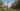The average SAT score of 1240 breaks down into:

• SAT math: 620

The average ACT score for University of Nebraska at Lincoln is 25 and their acceptance rate is 79.6%.

## University of Nebraska Omaha SAT scores

The average SAT score for University of Nebraska Omaha is 1140.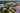The average SAT score of 1140 breaks down into:

• SAT math: 570

The average ACT score for University of Nebraska Omaha is 23 and their acceptance rate is 70.8%.

## Bryan College of Health Sciences SAT scores

The average SAT score for Bryan College of Health Sciences is 1110.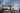The average SAT score of 1110 breaks down into:

• SAT math: 555

The average ACT score for Bryan College of Health Sciences is 22 and their acceptance rate is 40%.

## University of Nebraska at Kearney SAT scores

The average SAT score for University of Nebraska at Kearney is 1090.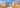The average SAT score of 1090 breaks down into:

• SAT math: 560

The average ACT score for University of Nebraska at Kearney is 22 and their acceptance rate is 81.7%.

## Union College SAT scores

The average SAT score for Union College is 1063.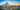The average SAT score of 1063 breaks down into:

• SAT math: 510

The average ACT score for Union College is 23 and their acceptance rate is 59.9%.

## Concordia University Nebraska SAT scores

The average SAT score for Concordia University Nebraska is 1017.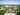The average SAT score of 1017 breaks down into:

• SAT math: 500